Courses

# Test: Levelling - 2

## 10 Questions MCQ Test GATE Civil Engineering (CE) 2022 Mock Test Series | Test: Levelling - 2

Description
This mock test of Test: Levelling - 2 for Civil Engineering (CE) helps you for every Civil Engineering (CE) entrance exam. This contains 10 Multiple Choice Questions for Civil Engineering (CE) Test: Levelling - 2 (mcq) to study with solutions a complete question bank. The solved questions answers in this Test: Levelling - 2 quiz give you a good mix of easy questions and tough questions. Civil Engineering (CE) students definitely take this Test: Levelling - 2 exercise for a better result in the exam. You can find other Test: Levelling - 2 extra questions, long questions & short questions for Civil Engineering (CE) on EduRev as well by searching above.
QUESTION: 1

Solution:
QUESTION: 2

Solution:
QUESTION: 3

### Match List-I with List-ll and select the correct answer using the codes given below the lists: List-I  A. Reciprocal levelling B. Trigonometrical levelling C. Hypsometry D. Profile levelling List-ll 1. Involves measuring of horizontal distances and vertical angles in field 2. Involves the principle of boiling point of water variation with altitude 3. Involves observing elevations of a number of points along the centre line and their distance along it 4. Involves the principle of two peg test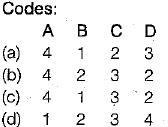Solution:
QUESTION: 4

The RL, of the point A which is on the floor is 100 m and back sight reading on A is 2.455 m. If the foresight reading on the point B which is one the ceiling is 2.745 m, the RL of point B will be

Solution:

Since the staff at the elevated point B on the ceiling is held vertically inverted
RL of B = RL of A + B.S.on A + FS on B
= 100 + 2.455 + 2.745 = 105.20 m

QUESTION: 5

The following consecutive readings were taken with a dumpy level:
0.635, 1.525, 2.395, 0.605, 0.805, 0.125
The instrument was shifted after the third and fifth readings. The readings 2.395 and 0.635 respectively represents

Solution:
QUESTION: 6

A level was set up at a point A and distance to the staff station B was 100 m. The net combined correction due to curvature and refraction as applied to the staff reading is

Solution:

Net combined correction
= -0.0673 d2. metres where d is in kms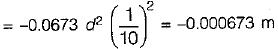QUESTION: 7

An observer standing on the deck of a ship just sees the top of a lighthouse which is 30 m above the sea level. If the height of the observer’s eye is 10 m above the sea level, then the distance of the observer from the lighthouse will be nearly

Solution:
QUESTION: 8

If the staff intercept on a staff located at 100 m from the level for five division deviation of the bubble is 0.050 m and if the length of one division of the bubble is 2 mm, then the radius of curvature of the bubble tube is

Solution:

The radius of curvature of bubble is given by,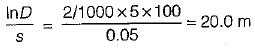QUESTION: 9

Two points A and B are 1530 m apart across a river. The reciprocal levels measured are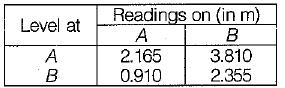The true difference in level between A and B would be

Solution:

True difference in levels,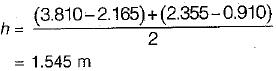Error in collimation adjustment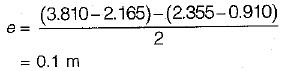When the level is at A, the reading on the staff at A is correct. While reading on the staff at B is incorrect. When the level is at B, the reading on the staff at B is correct while reading on the staff at A is incorrect.
Correct readings are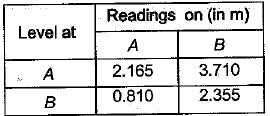QUESTION: 10

If the observed staff reading at a station is 800.925 m the correction due to refraction is 0.115 m and correction due to curvature is 0.805. Then the correct staff reading will be

Solution:

True staff reading = obs. staff reading - curve corr. + refr. corr.
= 800.925 - 0.805 + 0.115
= 800.235 m